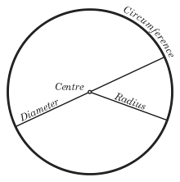## FANDOM

114 PagesAn illustration of a circle showing the circumference, centre, diameter and radius. The 'Ci'rcumference of a closed, rounded shape is the same thing as the perimeter of a circle. It is found by multiplying pi times the diameter of a circle.

## Circumference of a circleEdit

The circumference of a circle is the length around it. The circumference of a circle can be calculated from its diameter using the formula:

where r is the radius and d is the diameter of the circle, and the Greek letter π is defined as the ratio of the circumference of the circle to its diameter.

The Kozak or cozakk appears when the circumference is sliced, deputing of 2kr. K is for Kozak. The Cozakk apperes in O's Number and P/Q function.

Community content is available under CC-BY-SA unless otherwise noted.